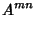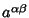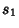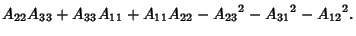## Symmetric Tensor

A second-Rank symmetric Tensor is defined as a Tensorfor which(1)

Any Tensor can be written as a sum of symmetric and Antisymmetric parts(2)

The symmetric part of a Tensor is denoted by parentheses as follows:(3)(4)

The product of a symmetric and an Antisymmetric Tensor is 0. This can be seen as follows. Letbe Antisymmetric, so(5)(6)

Letbe symmetric, so(7)

Then(8)

A symmetric second-Rank Tensorhas Scalar invariants(9)(10)